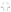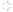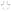# WARFRAME Wiki

Read about the future of the Fandom platform and the WARFRAME wiki! Any feedback would be appreciated! User blog:Cephalon Scientia/2022 Fandom Community Connect. - 06:11, 6 June 2022 (UTC)

Default Key Bindings for WARFRAME on all platforms. Key bindings can be changed in the Settings under the Controls tab.

## PC and Keyboard

These commands are for a standard QWERTY keyboard layout with the standard download. Steam users may find a few differences. For example, the screenshot key will place a picture of the game (without the in mission UI) to the "captures" folder: `C:\Program Files (x86)\Warframe\Downloaded\Public\Captures` for standard users. Screenshots taken this way contain metadata that will be helpful to developers to troubleshoot the game. Steam users will find screenshots in the "captures" folder: `C:\Program Files (x86)\Steam\SteamApps\common\Warframe\Captures`. Sometimes they are saved in `C:\Users\<Username>\Pictures\Warframe`.

Actions not listed below have no default key bindings set.

Action Default Key Binding Alternate Key Binding
Take Screenshot F6 (Can't Change)
Use Cipher Y (Can't Change)
Jump Space
Move Forward WMove Left AMove Backward SMove Right DMove Up (Archwing) Space
Move Down (Archwing) Ctrl
Fire Weapon Mouse button 1
Aim Weapon Mouse button 2
Secondary Fire Mouse button 3
Toggle Crouch V
Hold to Crouch Ctrl
Sprint/Roll Shift
Use X
Melee Attack E
Heavy Attack Mouse button 3  (If equipped)
Switch Weapon F
Gear Wheel Q
Chat T
Ability A 1 NUM 1
Ability B 2 NUM 2
Ability C 3 NUM 3
Ability D 4 NUM 4
Focus and Transference 5 NUM 5
Next Ability Mouse Wheel Up
Previous Ability Mouse Wheel Down
Place Marker/Captura Camera G
Reverse Camera H
Spectate Prev. Player O
Show Player List Z
Push to Talk C
Inspect I
Show Level Map M
View Mission Progress P
Quick Progress View Tab
Select Sub Gear 0 1  (in Gear wheel)
Select Sub Gear 1 2  (in Gear wheel)
Select Sub Gear 2 3  (in Gear wheel)
Available: F1-F5 , F7-F11 , 0 , 6-9 , NUM 0 , NUM 6-9 , NUM * , NUM - , NUM + , B , J , K , L , NU , ; , ' , \ , , , . , / , ` , - , E , Backspace , Insert , Delete , Home , End , Page Up , Page Down

Action Default Key Binding Alternate Key Binding
Check E
Ball Magnet Mouse button 2
Throw Mouse button 1
Pass R
Jump Space
Move Forward WMove Left AMove Backward SMove Right DToggle Crouch V
Hold to Crouch Ctrl
Sprint/Roll Shift
Use X
Gear Wheel Q
Chat T
Place Marker/Captura Camera G
Reverse Camera H
View Mission Progress P
Quick Progress View Tab

Action Default Key Binding Alternate Key Binding
Place Decoration 1
Modify Decoration/Place Another 2
Place Decoration Mouse button 1
Rotate Decoration Mouse button 2
Change Rotation Axis Z
Hold to scale R
Scale Up NUM +
Scale Down NUM -
Cycle Grid Snapping Shift
Surface Snapping/Free Placement F
Push/Pull decoration X
Move Forward WMove Left AMove Backward SMove Right DMove Up Space
Move Down Ctrl

Action Default Key Binding Alternate Key Binding
Jump WMove Left ACrouch SMove Right DLight Attack U
Medium Attack I
Heavy Attack O
Block J
Grab K
Special L

Action Default Key Binding Alternate Key Binding
Move Forward WMove Left AMove Backward SMove Right DMove Up Space
Move Down Ctrl
Boost/Maneuver Shift
Dismount X
Primary Fire Mouse button 1
Aim Weapon Mouse button 2
Ordnance Mouse button 3
Railjack Camera Right Ctrl

Action Default Key Binding Alternate Key Binding
1st String 1
2nd String 2
3rd String 3
Whammy Space
Sky FretEarth FretWater FretScale Tab
Songs W
Change Instrument A

### Configuration File

Main article: Key Bindings/Config

The configuration file for keybindings and other settings can be found in the following directory on Windows: `C:\Users\[Username]\AppData\Local\Warframe\EE.cfg`. Alternatively, one can go into the game's launcher and "Get Logs" to save the config file to `C:\Users\[Username]\Desktop\Warframe Logs`.

### Keybindings

Under `[LotusWindows_KeyBindings,/Lotus/Types/Input/KeyBindings]` in the configuration file.

Keybindings Key
Internal Name Context Default PC Binding
(English QWERTY)
`MOVE_X` Horizontal movement (left/right) `EN_D, EN_A, EN_RIGHT, EN_LEFT` `EN_GAMEPAD_LX`
`MOVE_Y` Vertical movement (up/down) `EN_LCONTROL, EN_SPACE` `EN_GAMEPAD_L2, EN_GAMEPAD_BUTTON_BOTTOM`
`MOVE_Z` Horizontal movement (forwards/backwards) `EN_S, EN_W, EN_DOWN, EN_UP` `EN_GAMEPAD_LY`
`SHOW_SECRET_1` Waypoint `EN_G` `EN_GAMEPAD_UP`
`PRE_ATTACK` Attacking `EN_MOUSE_B0` `EN_GAMEPAD_R1`
`RUN` Sprinting `EN_GAMEPAD_LTHUMB`
`ACTION` Rolling
`PRE_RUN` Sprinting & Rolling `EN_LSHIFT` `EN_GAMEPAD_LTHUMB`
`AIM_WEAPON` Aiming `EN_MOUSE_B1` `EN_GAMEPAD_L1`
`TROOPER_POWER` `EN_F` `EN_GAMEPAD_BUTTON_TOP`
`SECONDARY_FIRE` Secondary/Alternate Fire `EN_MOUSE_B2` `EN_GAMEPAD_RTHUMB`
`JUMP` Jumping `EN_SPACE` `EN_GAMEPAD_BUTTON_BOTTOM`
`USE` Interact `EN_X` `EN_GAMEPAD_BUTTON_LEFT`
`CYCLE_POWER_NEXT` Cycling abilities (left to right) `EN_MOUSE_WHEELUP` `EN_GAMEPAD_RIGHT`
`CYCLE_POWER_PREV` Cycling abilities (right to left) `EN_MOUSE_WHEELDOWN` `EN_GAMEPAD_LEFT`
`TOGGLE_CHAT_WINDOW` Opening Chat `EN_T`
`TOGGLE_CHAT_WINDOW_ALT` `EN_GAMEPAD_L1`
`SHOW_LEVEL_MAP` Opening map/enlarging minimap `EN_M` `EN_GAMEPAD_SELECT`
`VIEW_MISSION_PROGRESS` Toggle mission progress screen `EN_P`
`CYCLE_CAMERA_NEXT` Switching spectator camera
`CYCLE_CAMERA_PREV` Switching spectator camera `EN_O`
`REVERSE_CAMERA_OFFSET` Switch camera location `EN_H`
`NEXT_INV` Swapping weapons `EN_F` `EN_GAMEPAD_BUTTON_TOP`
`CROUCH` Toggle crouching `EN_V`
`HOLD_CROUCH` Crouching `EN_LCONTROL` `EN_GAMEPAD_L2`
`LEAN_LEFT` Rotate Railjack port-side `EN_Q`
`LEAN_RIGHT` Rotate Railjack starboard-side `EN_E`
`MELEE` Quick melee attack `EN_E` `EN_GAMEPAD_BUTTON_RIGHT`
`MELEE_SWING` Melee attack in melee mode `EN_E` `EN_GAMEPAD_BUTTON_RIGHT`
`MELEE_PARRY` Blocking `EN_MOUSE_B1` `EN_GAMEPAD_R1`
`MELEE_CHANNEL` Heavy Attack button `EN_MOUSE_B2` `EN_GAMEPAD_L1`
`RELOAD` Reloading `EN_R` `EN_GAMEPAD_BUTTON_LEFT`
`MINI_INVENTORY_HOLD` Gear menu `EN_Q`
`VIEW_HUMAN_PLAYERS` Toggle player list `EN_Z`
`ACTIVATE_ABILITY_0` First ability `EN_1, EN_KP_1`
`ACTIVATE_ABILITY_1` Second ability `EN_2, EN_KP_2`
`ACTIVATE_ABILITY_2` Third ability `EN_3, EN_KP_3`
`ACTIVATE_ABILITY_3` Fourth ability `EN_4, EN_KP_4`
`ACTIVATE_ABILITY_4` Transference `EN_5, EN_KP_5`
`PUSH_TO_TALK` Voice chat `EN_C`
`INSPECT` View player profile `EN_I` `EN_GAMEPAD_RIGHT`
`VIEW_QUICK_PROGRESS` View mission progress screen `EN_TAB`
`SELECT_SUB_GEAR_0` Equip fishing spear `EN_1`
`SELECT_SUB_GEAR_1` Use fish bait `EN_2`
`SELECT_SUB_GEAR_2` Use dye `EN_3`
`VIEW_RAILJACK_SYSTEMS` Open Railjack Tactical Menu `EN_L` `EN_GAMEPAD_LEFT`

### Other Settings

Note that settings that are not present in the config file are at its default value. Some default values are based on your system recommended settings as determined by the game.

Graphics and Other Settings Key
Internal Name Description Possible Values
`Stats.Visible` Framerate counter enabled 0 (off, default) or 1 (on)
`Sound.DeviceHwId` Sound device Device's Universally unique identifier
`Net.DTLS` Datagram Transport Layer Security enabled 0 or 1
`Graphics.AnisotropicFiltering` Anisotropic filtering `ATF_NONE, ATF_2X, ATF_4X, ATF_8X`
`Graphics.AntiAliasing` Temporal anti-aliasing `AA_DISABLED, AA_FXAA, AA_SMAA, AA_TEMPORAL`
`Graphics.AutoDetectGraphicsSettings` Auto detect graphic settings 0 or 1 (default)
`Graphics.BlurLocalReflections` Blur local reflections 0 or 1 (default)
`Graphics.Borderless` Borderless fullscreen 0 (default) or 1
`Graphics.BloomIntensity` Bloom intensity 0 to 1 (0.5 default)
`Graphics.Brightness` Brightness 0.1 to 1.9 (0.9 default)
`Graphics.ColorBlindCompensation` Colorblind filter `CBC_PROTANOPIA, CBC_DEUTERANOPIA, CBC_TRITANOPIA, CBC_NONE (default)`
`Graphics.ColorBlindCompensationStrength` Colorblind filter strength 0 to 100 (100 default)
`Graphics.Contrast` Contrast 0.25 to 1.75 (0.75 default)
`Graphics.DisplayAspectRatio` Display aspect ratio `FullScreen4x3, WideScreen16x9, WideScreen16x10, PanoramicWideScreen21x9, SuperUltraWideScreen32x9`
`Graphics.DynamicLighting` Dynamic lighting 0 or 1 (default)
`Graphics.DynamicResolution` Dynamic resolution `DYNRES_USER, DYNRES_AUTO`
`Graphics.EffectsIntensity` Visual effects intensity 0.1 to 2 (1 default)
`Graphics.EnableBloom` Bloom 0 or 1 (default)
`Graphics.EnableColorCorrection` Color correction 0 or 1 (default)
`Graphics.EnableContactShadows` Contact screen space shadows 0 (default) or 1
`Graphics.EnableDOF` Depth of field 0 or 1 (default)
`Graphics.EnableDistortions` Fullscreen distortions 0 or 1 (default)
`Graphics.EnableGlare` Glare 0 or 1 (default)
`Graphics.EnableGrain` Film grain 0 or 1 (default)
`Graphics.EnableMotionBlur` Motion blur 0 or 1 (default)
`Graphics.EnableScreenShake` Screen shake 0 or 1 (default)
`Graphics.EnableSwapChainFlipModel` Optimized swap chain flip model for Windows 10 0 (default) or 1
`Graphics.EnableVolumetricLighting` Volumetric Lighting 0 or 1 (default)
`Graphics.FoliageDistanceScale` Foilage level of detail 0 to 1
`Graphics.FullScreen` Fullscreen 0 (default) or 1
`Graphics.FullScreenSizeX` Length of fullscreen window (in pixels) Positive integer value
`Graphics.FullScreenSizeY` Width of fullscreen window (in pixels) Positive integer value
`Graphics.GPUParticlesQuality` GPU particles quality `GPQ_LOW, GPQ_MEDIUM, GPQ_HIGH, GPQ_LUDICROUS`
`Graphics.GeometryDetail` Geometry detail `GD_LOW, GD_MEDIUM, GD_HIGH`
`Graphics.HLODDistanceScale` Hierarchical level of detail (for large environments) 0 to 1
`Graphics.HighDPI` Window scaling mode (Dots per inch) `HDM_NATIVE, HDM_SCALED (default)`
`Graphics.HybridShadows` Character shadows 0 or 1 (default)
`Graphics.ImposterDistanceScale` Character avatar level of detail 0.5
`Graphics.LocalReflections` Local reflections 0 or 1 (default)
`Graphics.LowShaderQuality` Low shader quality 0 (default) or 1
`Graphics.MaxFrameRate` Maximum frame rate Positive integer value
`Graphics.ParticleSysQuality` Particle density `PQ_LOW, PQ_MEDIUM, PQ_HIGH`
`Graphics.ReduceTeamEffects` Reduce teammates' visual effects 0 (default) or 1
`Graphics.RefreshRate` Refresh rate Positive integer value
`Graphics.ResolutionScale` Dynamic resolution scale 0.5 to 1 (1 default)
`Graphics.SSAO` Screen space ambient occlusion 0 or 1 (default)
`Graphics.ShadowQuality` Shadow quality `SQ_LOW, SQ_MEDIUM, SQ_HIGH`
`Graphics.TAASharpen` Sharpening temporal anti-aliasing 0 to 100 (50 default)
`Graphics.TextureQuality` Texture quality `TQ_LOW, TQ_MEDIUM, TQ_HIGH`
`Graphics.TrilinearFiltering` Trilinear filtering `TF_OFF, TF_ON, TF_DEFAULT (default)`
`Graphics.UseDeferred` Deferred rendering 0 (default) or 1
`Graphics.UseDynamicDecals` Overlaying decal textures (e.g. bullet holes and blood splatters) 0 or 1 (default)
`Graphics.VSyncMode` Vertical synchronization `VSM_ALWAYS_SYNC, VSM_AUTO, VSM_NEVER_SYNC`
`Gamepad.LeftAnalogStickDeadzoneLow` Deadzone for gamepad left analog 0 to 1
`Gamepad.RightAnalogStickDeadzoneLow` Deadzone for gamepad right analog 0 to 1
`Gamepad.TriggerReleaseTransition` Gamepad trigger sensitivity -1 to 1
`Flash.FlashDrawScale` 0 to 1
`Flash.FlashDrawScaleMode` `MSM_CUSTOM`
`Client.Email` WARFRAME account email address Valid email address

### Example

Sample file:

View List
```+nowarning
+version=5

[KeyBindings,/EE/Types/Input/DefaultKeyBindings]

[LotusDedicatedServerAccountSettings,/Lotus/Types/Game/DedicatedServerAccountSettings]
email=example@email.com

[LotusDedicatedServerSettings,/Lotus/Types/Game/DedicatedServerSettings]
version=1
email=example@email.com
starChart=/Lotus/Types/Game/SolarMap/OriginSolarMapRedux
gameConfig=/Lotus/Types/GameRules/LotusDedicatedServerGameConfig
missionNode=PvpNode11
gameModeId=406011
eloRating=2
levelOverrides={
{
missionTag=PvpNode0
levels=/Lotus/Types/GameRules/PVPCTFLevels
},
{
missionTag=PvpNode10
levels=/Lotus/Types/GameRules/PVPDMLevels
},
{
missionTag=PvpNode9
levels=/Lotus/Types/GameRules/PVPDMLevels
},
{
missionTag=PvpNode11
levels=/Lotus/Types/GameRules/PVPSBLevels
}
}

[LotusWindows_KeyBindings,/Lotus/Types/Input/KeyBindings]
MOVE_X={
{
key=EN_D
inputMode=IM_NORMAL
invert=0
scale=1
inputFilter=/Lotus/Types/Player/TennoInputFilter
strictFiltering=0
slot=0
},
{
key=EN_RIGHT
inputMode=IM_NORMAL
invert=0
scale=1
inputFilter=/Lotus/Types/Player/TennoInputFilter
strictFiltering=0
slot=1
},
{
key=EN_D
inputMode=IM_NORMAL
invert=0
scale=1
inputFilter=/Lotus/Types/Player/TennoScoopEquippedMeleeFilter
strictFiltering=1
slot=0
},
{
key=EN_RIGHT
inputMode=IM_NORMAL
invert=0
scale=1
inputFilter=/Lotus/Types/Player/TennoScoopEquippedMeleeFilter
strictFiltering=1
slot=1
},
{
key=EN_D
inputMode=IM_NORMAL
invert=0
scale=1
inputFilter=/Lotus/Types/Game/CrewShip/RailJack/Input/RailjackInputFilter
strictFiltering=1
slot=0
},
{
key=EN_RIGHT
inputMode=IM_NORMAL
invert=0
scale=1
inputFilter=/Lotus/Types/Game/CrewShip/RailJack/Input/RailjackInputFilter
strictFiltering=1
slot=1
},
{
key=EN_A
inputMode=IM_NORMAL
invert=1
scale=1
inputFilter=/Lotus/Types/Player/TennoInputFilter
strictFiltering=0
slot=0
},
{
key=EN_LEFT
inputMode=IM_NORMAL
invert=1
scale=1
inputFilter=/Lotus/Types/Player/TennoInputFilter
strictFiltering=0
slot=1
},
{
key=EN_A
inputMode=IM_NORMAL
invert=1
scale=1
inputFilter=/Lotus/Types/Player/TennoScoopEquippedMeleeFilter
strictFiltering=1
slot=0
},
{
key=EN_LEFT
inputMode=IM_NORMAL
invert=1
scale=1
inputFilter=/Lotus/Types/Player/TennoScoopEquippedMeleeFilter
strictFiltering=1
slot=1
},
{
key=EN_A
inputMode=IM_NORMAL
invert=1
scale=1
inputFilter=/Lotus/Types/Game/CrewShip/RailJack/Input/RailjackInputFilter
strictFiltering=1
slot=0
},
{
key=EN_LEFT
inputMode=IM_NORMAL
invert=1
scale=1
inputFilter=/Lotus/Types/Game/CrewShip/RailJack/Input/RailjackInputFilter
strictFiltering=1
slot=1
},
{
inputMode=IM_NORMAL
invert=0
scale=1
inputFilter=""
strictFiltering=0
slot=0
}
}
MOVE_Y={
{
key=EN_LCONTROL
inputMode=IM_NORMAL
invert=1
scale=1
inputFilter=/Lotus/Types/Player/SpaceBattle/SpaceTennoInputFilter
strictFiltering=0
slot=0
},
{
key=EN_LCONTROL
inputMode=IM_NORMAL
invert=1
scale=1
inputFilter=/Lotus/Types/Game/CrewShip/RailJack/Input/RailjackInputFilter
strictFiltering=1
slot=0
},
{
key=EN_SPACE
inputMode=IM_NORMAL
invert=0
scale=1
inputFilter=/Lotus/Types/Player/SpaceBattle/SpaceTennoInputFilter
strictFiltering=0
slot=0
},
{
key=EN_SPACE
inputMode=IM_NORMAL
invert=0
scale=1
inputFilter=/Lotus/Types/Game/CrewShip/RailJack/Input/RailjackInputFilter
strictFiltering=1
slot=0
},
{
inputMode=IM_NORMAL
invert=0
scale=1
inputFilter=""
strictFiltering=0
slot=0
},
{
inputMode=IM_NORMAL
invert=1
scale=1
inputFilter=""
strictFiltering=0
slot=0
}
}
MOVE_Z={
{
key=EN_S
inputMode=IM_NORMAL
invert=1
scale=1
inputFilter=/Lotus/Types/Player/TennoInputFilter
strictFiltering=0
slot=0
},
{
key=EN_DOWN
inputMode=IM_NORMAL
invert=1
scale=1
inputFilter=/Lotus/Types/Player/TennoInputFilter
strictFiltering=0
slot=1
},
{
key=EN_DOWN
inputMode=IM_NORMAL
invert=1
scale=1
inputFilter=/Lotus/Types/Player/TennoScoopEquippedMeleeFilter
strictFiltering=1
slot=0
},
{
key=EN_S
inputMode=IM_NORMAL
invert=1
scale=1
inputFilter=/Lotus/Types/Game/CrewShip/RailJack/Input/RailjackInputFilter
strictFiltering=1
slot=0
},
{
key=EN_DOWN
inputMode=IM_NORMAL
invert=1
scale=1
inputFilter=/Lotus/Types/Game/CrewShip/RailJack/Input/RailjackInputFilter
strictFiltering=1
slot=1
},
{
key=EN_W
inputMode=IM_NORMAL
invert=0
scale=1
inputFilter=/Lotus/Types/Player/TennoInputFilter
strictFiltering=0
slot=0
},
{
key=EN_UP
inputMode=IM_NORMAL
invert=0
scale=1
inputFilter=/Lotus/Types/Player/TennoInputFilter
strictFiltering=0
slot=1
},
{
key=EN_W
inputMode=IM_NORMAL
invert=0
scale=1
inputFilter=/Lotus/Types/Player/TennoScoopEquippedMeleeFilter
strictFiltering=1
slot=0
},
{
key=EN_UP
inputMode=IM_NORMAL
invert=0
scale=1
inputFilter=/Lotus/Types/Player/TennoScoopEquippedMeleeFilter
strictFiltering=1
slot=1
},
{
key=EN_W
inputMode=IM_NORMAL
invert=0
scale=1
inputFilter=/Lotus/Types/Game/CrewShip/RailJack/Input/RailjackInputFilter
strictFiltering=1
slot=0
},
{
key=EN_UP
inputMode=IM_NORMAL
invert=0
scale=1
inputFilter=/Lotus/Types/Game/CrewShip/RailJack/Input/RailjackInputFilter
strictFiltering=1
slot=1
},
{
inputMode=IM_NORMAL
invert=1
scale=1
inputFilter=""
strictFiltering=0
slot=0
}
}
SHOW_SECRET_1={
{
key=EN_G
inputMode=IM_NORMAL
invert=0
scale=1
inputFilter=/Lotus/Types/Player/TennoInputFilter
strictFiltering=0
slot=0
},
{
key=EN_G
inputMode=IM_NORMAL
invert=0
scale=1
inputFilter=/Lotus/Types/Player/TennoScoopEquippedMeleeFilter
strictFiltering=1
slot=0
},
{
inputMode=IM_NORMAL
invert=0
scale=1
inputFilter=""
strictFiltering=0
slot=0
}
}
PRE_ATTACK={
{
key=EN_MOUSE_B0
inputMode=IM_NORMAL
invert=0
scale=1
inputFilter=/Lotus/Types/Player/TennoInputFilter
strictFiltering=0
slot=0
},
{
key=EN_MOUSE_B0
inputMode=IM_NORMAL
invert=0
scale=1
inputFilter=/Lotus/Types/Game/CrewShip/RailJack/Input/RailjackInputFilter
strictFiltering=1
slot=0
},
{
inputMode=IM_NORMAL
invert=0
scale=1
inputFilter=""
strictFiltering=0
slot=0
}
}
RUN={
{
inputMode=IM_NORMAL
invert=0
scale=1
inputFilter=""
strictFiltering=0
slot=0
},
{
inputMode=IM_NORMAL
invert=0
scale=1
inputFilter=""
strictFiltering=1
slot=0
}
}
PRE_RUN={
{
key=EN_LSHIFT
inputMode=IM_NORMAL
invert=0
scale=1
inputFilter=/Lotus/Types/Player/TennoInputFilter
strictFiltering=0
slot=0
},
{
key=EN_LSHIFT
inputMode=IM_NORMAL
invert=0
scale=1
inputFilter=/Lotus/Types/Player/TennoScoopEquippedMeleeFilter
strictFiltering=1
slot=0
},
{
key=EN_LSHIFT
inputMode=IM_NORMAL
invert=0
scale=1
inputFilter=/Lotus/Types/Game/CrewShip/RailJack/Input/RailjackInputFilter
strictFiltering=1
slot=0
},
{
inputMode=IM_NORMAL
invert=0
scale=1
inputFilter=""
strictFiltering=0
slot=0
}
}
AIM_WEAPON={
{
key=EN_MOUSE_B1
inputMode=IM_NORMAL
invert=0
scale=1
inputFilter=/Lotus/Types/Player/TennoInputFilter
strictFiltering=0
slot=0
},
{
key=EN_MOUSE_B1
inputMode=IM_NORMAL
invert=0
scale=1
inputFilter=/Lotus/Types/Game/CrewShip/RailJack/Input/RailjackInputFilter
strictFiltering=1
slot=0
},
{
inputMode=IM_NORMAL
invert=0
scale=1
inputFilter=""
strictFiltering=0
slot=0
}
}
TROOPER_POWER={
{
key=EN_F
inputMode=IM_NORMAL
invert=0
scale=1
inputFilter=/Lotus/Types/Game/CrewShip/RailJack/Input/RailjackInputFilter
strictFiltering=1
slot=0
},
{
inputMode=IM_NORMAL
invert=0
scale=1
inputFilter=/Lotus/Types/Game/CrewShip/RailJack/Input/RailjackInputFilter
strictFiltering=0
slot=0
}
}
SECONDARY_FIRE={
{
key=EN_LALT
inputMode=IM_NORMAL
invert=0
scale=1
inputFilter=/Lotus/Types/Player/TennoInputFilter
strictFiltering=0
slot=0
},
{
inputMode=IM_NORMAL
invert=0
scale=1
inputFilter=""
strictFiltering=0
slot=0
},
{
key=EN_LALT
inputMode=IM_NORMAL
invert=0
scale=1
inputFilter=/Lotus/Types/Game/CrewShip/RailJack/Input/RailjackInputFilter
strictFiltering=1
slot=0
}
}
JUMP={
{
key=EN_SPACE
inputMode=IM_NORMAL
invert=0
scale=1
inputFilter=/Lotus/Types/Player/TennoInputFilter
strictFiltering=0
slot=0
},
{
key=EN_SPACE
inputMode=IM_NORMAL
invert=0
scale=1
inputFilter=/Lotus/Types/Player/TennoScoopEquippedMeleeFilter
strictFiltering=1
slot=0
},
{
inputMode=IM_NORMAL
invert=0
scale=1
inputFilter=""
strictFiltering=0
slot=0
}
}
USE={
{
key=EN_X
inputMode=IM_NORMAL
invert=0
scale=1
inputFilter=/Lotus/Types/Player/TennoInputFilter
strictFiltering=0
slot=0
},
{
key=EN_X
inputMode=IM_NORMAL
invert=0
scale=1
inputFilter=/Lotus/Types/Player/TennoScoopEquippedMeleeFilter
strictFiltering=1
slot=0
},
{
key=EN_X
inputMode=IM_NORMAL
invert=0
scale=1
inputFilter=/Lotus/Types/Game/CrewShip/RailJack/Input/RailjackInputFilter
strictFiltering=1
slot=0
},
{
inputMode=IM_NORMAL
invert=0
scale=1
inputFilter=""
strictFiltering=0
slot=0
}
}
CYCLE_POWER_NEXT={
{
key=EN_MOUSE_WHEELUP
inputMode=IM_NORMAL
invert=0
scale=1
inputFilter=/Lotus/Types/Player/TennoInputFilter
strictFiltering=0
slot=0
},
{
inputMode=IM_NORMAL
invert=0
scale=1
inputFilter=""
strictFiltering=0
slot=0
}
}
CYCLE_POWER_PREV={
{
inputMode=IM_NORMAL
invert=0
scale=1
inputFilter=""
strictFiltering=0
slot=0
}
}
TOGGLE_CHAT_WINDOW={
{
key=EN_T
inputMode=IM_NORMAL
invert=0
scale=1
inputFilter=/Lotus/Types/Player/TennoInputFilter
strictFiltering=0
slot=0
},
{
key=EN_T
inputMode=IM_NORMAL
invert=0
scale=1
inputFilter=/Lotus/Types/Player/TennoScoopEquippedMeleeFilter
strictFiltering=1
slot=0
}
}
TOGGLE_CHAT_WINDOW_ALT={
{
inputMode=IM_NORMAL
invert=0
scale=1
strictFiltering=0
slot=0
}
}
SHOW_LEVEL_MAP={
{
key=EN_M
inputMode=IM_NORMAL
invert=0
scale=1
inputFilter=/Lotus/Types/Player/TennoInputFilter
strictFiltering=0
slot=0
},
{
inputMode=IM_NORMAL
invert=0
scale=1
inputFilter=""
strictFiltering=0
slot=0
}
}
VIEW_MISSION_PROGRESS={
{
key=EN_P
inputMode=IM_NORMAL
invert=0
scale=1
inputFilter=/Lotus/Types/Player/TennoInputFilter
strictFiltering=0
slot=0
},
{
key=EN_P
inputMode=IM_NORMAL
invert=0
scale=1
inputFilter=/Lotus/Types/Player/TennoScoopEquippedMeleeFilter
strictFiltering=1
slot=0
}
}
CYCLE_CAMERA_NEXT={}
CYCLE_CAMERA_PREV={
{
key=EN_O
inputMode=IM_NORMAL
invert=0
scale=1
inputFilter=/Lotus/Types/Player/TennoInputFilter
strictFiltering=0
slot=0
}
}
REVERSE_CAMERA_OFFSET={
{
key=EN_H
inputMode=IM_NORMAL
invert=0
scale=1
inputFilter=/Lotus/Types/Player/TennoInputFilter
strictFiltering=0
slot=0
},
{
key=EN_H
inputMode=IM_NORMAL
invert=0
scale=1
inputFilter=/Lotus/Types/Player/TennoScoopEquippedMeleeFilter
strictFiltering=1
slot=0
}
}
NEXT_INV={
{
key=EN_F
inputMode=IM_NORMAL
invert=0
scale=1
inputFilter=/Lotus/Types/Player/TennoInputFilter
strictFiltering=0
slot=0
},
{
inputMode=IM_NORMAL
invert=0
scale=1
inputFilter=""
strictFiltering=0
slot=0
}
}
CROUCH={
{
key=EN_V
inputMode=IM_NORMAL
invert=0
scale=1
inputFilter=/Lotus/Types/Player/TennoInputFilter
strictFiltering=0
slot=0
},
{
key=EN_V
inputMode=IM_NORMAL
invert=0
scale=1
inputFilter=/Lotus/Types/Player/TennoScoopEquippedMeleeFilter
strictFiltering=1
slot=0
}
}
HOLD_CROUCH={
{
key=EN_LCONTROL
inputMode=IM_NORMAL
invert=0
scale=1
inputFilter=/Lotus/Types/Player/TennoInputFilter
strictFiltering=0
slot=0
},
{
key=EN_LCONTROL
inputMode=IM_NORMAL
invert=0
scale=1
inputFilter=/Lotus/Types/Player/TennoScoopEquippedMeleeFilter
strictFiltering=1
slot=0
},
{
inputMode=IM_NORMAL
invert=0
scale=1
inputFilter=""
strictFiltering=0
slot=0
}
}
LEAN_LEFT={
{
key=EN_Q
inputMode=IM_NORMAL
invert=0
scale=1
inputFilter=/Lotus/Types/Game/CrewShip/RailJack/Input/RailjackInputFilter
strictFiltering=1
slot=0
},
{
inputMode=IM_NORMAL
invert=0
scale=1
inputFilter=/Lotus/Types/Game/CrewShip/RailJack/Input/RailjackInputFilter
strictFiltering=1
slot=0
}
}
LEAN_RIGHT={
{
key=EN_E
inputMode=IM_NORMAL
invert=0
scale=1
inputFilter=/Lotus/Types/Game/CrewShip/RailJack/Input/RailjackInputFilter
strictFiltering=1
slot=0
}
}
MELEE={
{
key=EN_E
inputMode=IM_NORMAL
invert=0
scale=1
inputFilter=/Lotus/Types/Player/TennoInputFilter
strictFiltering=0
slot=0
},
{
inputMode=IM_NORMAL
invert=0
scale=1
inputFilter=""
strictFiltering=0
slot=0
}
}
MELEE_SWING={
{
key=EN_E
inputMode=IM_NORMAL
invert=0
scale=1
inputFilter=/Lotus/Types/Player/TennoEquippedMeleeFilter
strictFiltering=0
slot=0
},
{
inputMode=IM_NORMAL
invert=0
scale=1
inputFilter=""
strictFiltering=0
slot=0
}
}
MELEE_PARRY={
{
key=EN_MOUSE_B1
inputMode=IM_NORMAL
invert=0
scale=1
inputFilter=/Lotus/Types/Player/TennoEquippedMeleeFilter
strictFiltering=0
slot=0
},
{
inputMode=IM_NORMAL
invert=0
scale=1
inputFilter=""
strictFiltering=0
slot=0
}
}
MELEE_CHANNEL={
{
key=EN_LALT
inputMode=IM_NORMAL
invert=0
scale=1
inputFilter=/Lotus/Types/Player/TennoEquippedMeleeFilter
strictFiltering=0
slot=0
},
{
inputMode=IM_NORMAL
invert=0
scale=1
inputFilter=""
strictFiltering=0
slot=0
}
}
{
key=EN_R
inputMode=IM_NORMAL
invert=0
scale=1
inputFilter=/Lotus/Types/Player/TennoInputFilter
strictFiltering=0
slot=0
},
{
inputMode=IM_NORMAL
invert=0
scale=1
inputFilter=""
strictFiltering=0
slot=0
}
}
MINI_INVENTORY_HOLD={
{
key=EN_Q
inputMode=IM_NORMAL
invert=0
scale=1
inputFilter=/Lotus/Types/Player/TennoInputFilter
strictFiltering=0
slot=0
},
{
key=EN_Q
inputMode=IM_NORMAL
invert=0
scale=1
inputFilter=/Lotus/Types/Player/TennoScoopEquippedMeleeFilter
strictFiltering=1
slot=0
}
}
VIEW_HUMAN_PLAYERS={
{
key=EN_Z
inputMode=IM_NORMAL
invert=0
scale=1
inputFilter=/Lotus/Types/Player/TennoInputFilter
strictFiltering=0
slot=0
}
}
ACTIVATE_ABILITY_0={
{
key=EN_1
inputMode=IM_NORMAL
invert=0
scale=1
inputFilter=/Lotus/Types/Player/TennoInputFilter
strictFiltering=0
slot=0
},
{
key=EN_KP_1
inputMode=IM_NORMAL
invert=0
scale=1
inputFilter=/Lotus/Types/Player/TennoInputFilter
strictFiltering=0
slot=1
}
}
ACTIVATE_ABILITY_1={
{
key=EN_2
inputMode=IM_NORMAL
invert=0
scale=1
inputFilter=/Lotus/Types/Player/TennoInputFilter
strictFiltering=0
slot=0
},
{
key=EN_KP_2
inputMode=IM_NORMAL
invert=0
scale=1
inputFilter=/Lotus/Types/Player/TennoInputFilter
strictFiltering=0
slot=1
}
}
ACTIVATE_ABILITY_2={
{
key=EN_3
inputMode=IM_NORMAL
invert=0
scale=1
inputFilter=/Lotus/Types/Player/TennoInputFilter
strictFiltering=0
slot=0
},
{
key=EN_KP_3
inputMode=IM_NORMAL
invert=0
scale=1
inputFilter=/Lotus/Types/Player/TennoInputFilter
strictFiltering=0
slot=1
}
}
ACTIVATE_ABILITY_3={
{
key=EN_4
inputMode=IM_NORMAL
invert=0
scale=1
inputFilter=/Lotus/Types/Player/TennoInputFilter
strictFiltering=0
slot=0
},
{
key=EN_KP_4
inputMode=IM_NORMAL
invert=0
scale=1
inputFilter=/Lotus/Types/Player/TennoInputFilter
strictFiltering=0
slot=1
}
}
ACTIVATE_ABILITY_4={
{
key=EN_5
inputMode=IM_NORMAL
invert=0
scale=1
inputFilter=/Lotus/Types/Player/TennoInputFilter
strictFiltering=0
slot=0
},
{
key=EN_KP_5
inputMode=IM_NORMAL
invert=0
scale=1
inputFilter=/Lotus/Types/Player/TennoInputFilter
strictFiltering=0
slot=1
}
}
PUSH_TO_TALK={
{
key=EN_C
inputMode=IM_NORMAL
invert=0
scale=1
inputFilter=/Lotus/Types/Player/TennoInputFilter
strictFiltering=0
slot=0
}
}
INSPECT={
{
key=EN_I
inputMode=IM_NORMAL
invert=0
scale=1
inputFilter=/Lotus/Types/Player/TennoInputFilter
strictFiltering=0
slot=0
},
{
inputMode=IM_NORMAL
invert=0
scale=1
inputFilter=""
strictFiltering=0
slot=0
}
}
VIEW_QUICK_PROGRESS={
{
key=EN_TAB
inputMode=IM_NORMAL
invert=0
scale=1
inputFilter=/Lotus/Types/Player/TennoInputFilter
strictFiltering=0
slot=0
},
{
key=EN_TAB
inputMode=IM_NORMAL
invert=0
scale=1
inputFilter=/Lotus/Types/Player/TennoScoopEquippedMeleeFilter
strictFiltering=1
slot=0
}
}
SELECT_SUB_GEAR_0={
{
key=EN_1
inputMode=IM_NORMAL
invert=0
scale=1
inputFilter=/Lotus/Types/Player/TennoSpearFishingInputFilter
strictFiltering=0
slot=0
}
}
SELECT_SUB_GEAR_1={
{
key=EN_2
inputMode=IM_NORMAL
invert=0
scale=1
inputFilter=/Lotus/Types/Player/TennoSpearFishingInputFilter
strictFiltering=0
slot=0
}
}
SELECT_SUB_GEAR_2={
{
key=EN_3
inputMode=IM_NORMAL
invert=0
scale=1
inputFilter=/Lotus/Types/Player/TennoSpearFishingInputFilter
strictFiltering=0
slot=0
}
}
VIEW_RAILJACK_SYSTEMS={
{
key=EN_L
inputMode=IM_NORMAL
invert=0
scale=1
inputFilter=/Lotus/Types/Game/CrewShip/RailJack/Input/RailjackInputFilter
strictFiltering=0
slot=0
},
{
inputMode=IM_NORMAL
invert=0
scale=1
inputFilter=""
strictFiltering=0
slot=0
}
}

[Windows_Config,/EE/Types/Base/Config]
Stats.Visible=1
Sound.DeviceHwId="{0.0.0.00000000}.{974b0233-39c3-4995-9f91-68e2e4c3e1be}"
Net.DTLS=1
Graphics.AutoDetectGraphicsSettings=0
Graphics.Borderless=1
Graphics.Brightness=1.018
Graphics.DisplayAspectRatio=WideScreen16x9
Graphics.EnableBloom=0
Graphics.EnableColorCorrection=0
Graphics.EnableDOF=0
Graphics.EnableGlare=0
Graphics.EnableGrain=0
Graphics.EnableMotionBlur=0
Graphics.EnableSwapChainFlipModel=1
Graphics.FullScreenSizeX=1920
Graphics.FullScreenSizeY=1080
Graphics.GPUParticlesQuality=GPQ_MEDIUM
Graphics.GeometryDetail=GD_HIGH
Graphics.HighDPI=HDM_NATIVE
Graphics.MaxFrameRate=60
Graphics.ReduceTeamEffects=1
Graphics.SSAO=0
Graphics.TextureQuality=TQ_HIGH
Graphics.TrilinearFiltering=TF_ON
Graphics.UseDeferred=1
Graphics.UseDynamicDecals=0
Graphics.VSyncMode=VSM_NEVER_SYNC
Client.Email=example@email.com
```

## Playstation

1. Use left joystick to move.
2. Toggle sprint by pressing down the left joystick L3 .
3. Control camera by moving the right joystick.
4. Use equipped weapon's secondary fire or perform a Melee Heavy Attack by pressing down the right joystick R3 .
5. Pressto jump.
6. Pressto perform a quick melee attack.
• Use while in melee-only mode to melee attack.
7. Pressto reload your weapon or perform context actions.
8. Pressto switch weapons.
• Hold to switch to melee-only mode.
• Press Up to place a Waypoint marker.
• Press Down to access your Gear wheel and emotes.
• Press Left/Right to cycle through your Warframe's abilities.
10. Hold L1  to crouch when standing still.
• Hold while moving to slide.
• Press while moving to roll.
11. Hold L2  to take aim and zoom in.
12. Press R1  button to open the Ability menu.
13. Press R2  to fire your weapon.
• Use while in melee-only mode to block attacks.

## Xbox

1. Use left joystick to move.
2. Toggle sprint by pressing down the left joystick.
3. Control camera by moving the right joystick.
4. Use equipped weapon's secondary fire or perform a Melee Heavy Attack by pressing down the right joystick.
5. Pressto jump.
6. Pressto perform a quick melee attack.
• Use while in melee-only mode to melee attack.
7. Pressto reload your weapon or perform context actions.
8. Pressto switch weapons.
• Hold to switch to melee-only mode.
• Press Up to place a Waypoint marker.
• Press Down to access your Gear wheel and emotes.
• Press Left/Right to cycle through your Warframe's abilities.
10. Press LB  to crouch when standing still.
• Use while moving to roll.
• Use while running to slide.
11. Hold LT  to take aim and zoom in.
12. Press RB  button to open the Ability menu.
13. Press RT  to fire your weapon.
• Use while in melee-only mode to block attacks.

## Nintendo Switch

1. Use left joystick to move.
2. Toggle sprint by pressing down the left joystick.
3. Control camera by moving the right joystick.
4. Use equipped weapon's secondary fire or perform a Melee Heavy Attack by pressing down the right joystick.
5. Press B  to jump.
6. Press A  to perform a quick melee attack.
• Use while in melee-only mode to melee attack.
8. Press Y  to switch weapons.
• Hold to switch to melee-only mode.